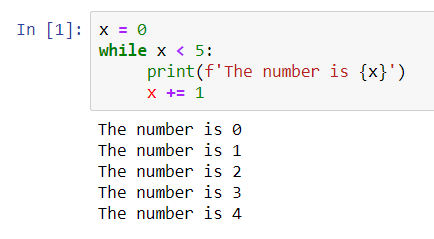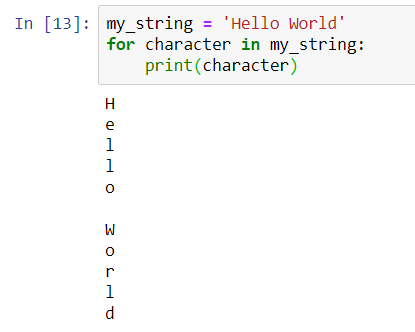# Python: Introduction III continue from the Python: Introduction which I wrote it yesterday and it gave the very basic idea of how Python is in term of declaring variables, the data types and how we can store the data in a collection. So. variable, data type and collections. If you missed out or cannot recall them, here is the link to yesterday’s post. There are links to various data types and the collections. I did not want to repeat here.

For the part 2, I have decided to concentrate on writing the introduction to control flows. I think it will be great to have these control flows being taught first before we head to more Data Science orientated topic such as using the package called Numpy and creating our own functions and methods. So, I swapped a little bit in term of syllabus or topic in my writing.

## if, elif, else

It is a conditional statement which we can use to match certain conditions. There are few methods to write this statement and it is not always that we need to use elif and else. Let us look at this syntax below:

```if condition :
expression
```

This syntax has 1 single condition to match only, that is why “if” is being used. Example:

```z = 4
if z % 2 == 0 :
print("z is even")
```

All control flows has a standard syntax and indentation applied to each of them to mark the beginning to the expression or what should it does when the condition is matched. Therefore, you can see the print() statement is slightly indented. In most IDEs, it is automatically indented when we use the colon (:) sign after the condition, in this case, z % 2 == 0.

The moment we have 1 more condition in our code, if else statement is used. See the syntax below:

```if condition :
expression
else :
expression
```

In the else statement, often we do not need to specify the condition within the line because it is understood that when the if statement or condition did not match then it goes to the else statement and execute the code. It looks as though else statement is a default statement. I know in some other programming language, it does have default statement at the end of else statement which say, anything not matches then run default statement.

We can omit this else statement when we do not require to process anything if the first condition does not match. However, sometimes, we might miss out important scenarios of the if statement is skipped. What I meant here is besides being legit that the condition does not match, there is also possibilities of exceptions happened while checking through the conditions. It is recommended to use the else statement as an exception handling, either print out a line in the console or log file. This helps in the debugging procedure.

```z = 5
if z % 2 == 0 :
print("z is even")
else
print("z is odd")
```

The above is an example of using if-else statement when there are two conditions, either-or situation. The variable z is 5, hence it goes to the else statement and print out “z is odd”.

Next, if-elif-else statement is used when there are few conditions in the scenario which one may get matched during the condition checking. When the first condition does not match, it goes to the next elif condition to check until it has no matches, it will end at else statement. You can have many elif statements in your codes. Below is the syntax:

```if condition :
expression
elif condition :
expression
else :
expression
```

Example of using the syntax:

```z = 3
if z % 2 == 0 :
print("z is divisible by 2")
elif z % 3 == 0 :
print("z is divisible by 3")
else :
print("z is neither divisible by 2 nor by 3")
```

The output is z is divisible by 3. After each expression, the statement terminates and returns the result. It will not proceed to check whether next condition is matched. With these 3 examples, I hope it gives some ideas about if statement, if-else statement and if-elif-else statement.

## while

while statement works by repeating an action until condition is met. It is important to assess the code before running the while statement because if any chances the condition is not met, the statement will keep running and this we call it infinite loop. You have to force to end the application manually. The syntax for while statement:

```while condition :
expression
```

Example of using the while syntax:

```x = 0
while x < 5:
print(f'The number is {x}')
x += 1
```

The most crucial part here is the variable x which works as a counter to ensure the condition is met. Without this line of code (x += 1 ) the condition is always true and the loop becomes infinite.This is the output from executing the while statement. When x = 5, it stops and exits from the while statement and does not print out anything.

## for

Remember in the previous blog, I mentioned about using Python list (collections)? For statement is a good control flow to iterate (repeat) through the Python list to get each element.

```for var in seq :
expression
```

Without using for statement, we might want to repeat few times of the print statements to print out the elements inside the Python list below:

```fam = [1.73, 1.68, 1.71, 1.89]

print(fam)
print(fam)
print(fam)
print(fam)
```

Although this is a correct syntax, it is not a good practice. Below demonstrates how to use a for statement to iterate through the Python list and print the values out.

```for height in fam :
print(height)
```

Both of the codes returns the same output.

```1.73
1.68
1.71
1.89
```

For statement works well for any types of collections or even with a string. Below example uses my_string as the “list” and a variable character as the “item_name” to represent the elements inside the list. One by one, it prints out each character in the my_string.In for statement, we can use the enumerate(), a Python built-in function. enumerate() method adds a counter to an iterable and returns it in a form of enumerate object. This enumerate object can then be used directly in for loops or be converted into a list of tuples using list() method.

### Why is enumerate return a tuple?

When enumerate() returns in a form of enumerate object, it comes in a form of index, value. It is because enumerate() accepts start parameter which is the index value of the counter, by default it is 0. A simple illustration is as below:

```enumerate(iterable, start=0)
```
```list = ["eat","sleep","repeat"]
print(enumerate(list)
```

When we check the output from the console, it shows as below:

```[(0, 'eat'), (1, 'sleep'), (2, 'repeat')]
```

It starts with an index 0, of course, it can be changed with indicating the index value, such as enumerate(list,1), then the index begins with 1 instead of 0. This enumerate() function may look useful when we want to list the elements from the collections with the index and value.

```fam = [1.73, 1.68, 1.71, 1.89]
for index, height in enumerate(fam) :
print("index " + str(index) + ": " + str(height))
```

Reusing the above example and now, we have added enumerate(fam) in the for statement instead of using “for height in fam”. Then, in the print() statement, we convert the index value and height value to string and concatenate them. This maybe useful when we want to print out our shopping cart’s items list. Its output shows:

```index 0: 1.73
index 1: 1.68
index 2: 1.71
index 3: 1.89
```

Mastering the use of the control flows can help in the later stage when we go into the data structure section. I have written separate blogs about if-else statements, while and for loops, you can refer to the links below: